• 怎样可以设置3D旋转的中心轴啊 比如我一张图片要以图片自己的中心为旋转，以一条X轴成320度，Y轴成-25度的中心轴旋转，就是做出类似3D星云旋转画面的效果，要怎么设置啊3d web前端 图片
• #coding:utf-8 import cv2 import numpy as np import imutils import matplotlib.pyplot as plt import os from multiprocessing import Pool,Process ...红点代表长轴点，黑点代表短轴。
#coding:utf-8
import cv2
import numpy as np
import imutils
import matplotlib.pyplot as plt
import os
from multiprocessing import Pool,Process
import time

def get_dis(A,B):
BT = np.transpose(B)
A_BT = np.dot(A, BT)
Asq = A ** 2
Asq = np.tile(np.sum(Asq, axis=1, keepdims=True), (1, A_BT.shape))
Bsq = BT ** 2
Bsq = np.tile(np.sum(Bsq, axis=0, keepdims=True), (A_BT.shape, 1))
ED = np.sqrt(Asq + Bsq - 2 * A_BT)
# print('ED.shape',ED.shape)
long_axis_ind = np.unravel_index(np.argmax(ED), ED.shape)
return ED[long_axis_ind,long_axis_ind],long_axis_ind
def show_points(axis_ind,points1,points2,color):
i_ = axis_ind
j_ = axis_ind
x1, y1 = points1[i_, :]
x2, y2 = points2[j_, :]
plt.plot([x1, x2], [y1, y2], color=color)
return x1, y1,x2, y2
def get_contour_centroid(cnt):
m = cv2.moments(cnt)
c_x = int(m['m10'] / m['m00'])
c_y = int(m['m01'] / m['m00'])
return c_x, c_y

def get_line_sides(points,center_x):
side_points=[]
another_side_points = []
for point in points:
if point>center_x:
side_points.append(point)
else:
another_side_points.append(point)
return np.array(side_points),np.array(another_side_points)

def get_disk_axis(image_list_path):

# image_list_path='./data/sichuan_pig_mistake_label/2018-9-11-IMG_0319.jpg'

print(image_list_path)
img=cv2.cvtColor(img,cv2.COLOR_BGR2GRAY)
h,w=img.shape
image_thre = cv2.threshold(img, 127, 255, cv2.THRESH_BINARY)
cnts = cv2.findContours(image_thre, cv2.RETR_EXTERNAL, cv2.CHAIN_APPROX_SIMPLE)
cnts = cnts if imutils.is_cv2() else cnts
c = sorted(cnts, key=cv2.contourArea, reverse=True)
disk_cnt = np.squeeze(c)
# plt.plot(disk_cnt[:,0],disk_cnt[:,1])

y, x = np.where(dummy_mask[:, :, 0] == 255)

plt.figure()
plt.gca().set_aspect('equal')
disk_complet_points = np.stack((x, y), axis=-1)
center_x,center_y=get_contour_centroid(disk_cnt)
# print('center_x,center_y=',center_x,center_y)
plt.plot(disk_complet_points[:, 0], disk_complet_points[:, 1], 'o')
###
plt.scatter(center_x,center_y,color='black')

side_points,another_side_points=get_line_sides(disk_complet_points,center_x=center_x)
# print('disk_complet_points.shape',disk_complet_points.shape)
# print('side_points.shape',side_points.shape)
# print('another_side_points.shape', another_side_points.shape)
long_axis_length,long_axis_ind=get_dis(side_points,another_side_points)
print('long_axis_length=',long_axis_length)
x1, y1, x2, y2=show_points(long_axis_ind,side_points,another_side_points,color='red')

del_side_points = np.delete(side_points, obj=(long_axis_ind), axis=0)
del_another_side_points = np.delete(another_side_points, obj=(long_axis_ind), axis=0)
del_complet_points = np.vstack((del_side_points, del_another_side_points))

short_axis_points = []
if y2 - y1:
k= -(x2-x1)/(y2-y1)
b = center_y - k * center_x
print('k,b',k,b)
for point in del_complet_points:
if abs(np.ceil(point - k * point - b)) < 20:
short_axis_points.append(point)
else:
for point in del_complet_points:
if abs(center_x-point)<10:
short_axis_points.append(point)
short_side_points, short_another_side_points = get_line_sides(np.array(short_axis_points), center_x=center_x)

short_axis_length, short_axis_ind = get_dis(short_side_points, short_another_side_points)
_,_,_,_,=show_points(short_axis_ind,short_side_points, short_another_side_points,color='blue')

# plt.show()
#debug see output
if not os.path.exists(out_path):
os.mkdir(out_path)
plt.savefig(out_path+'/'+image_list_path.split('/')[-1])
def main():
start_time = time.time()
pool = Pool()
images_list_path=[os.path.join(path,i) for i in os.listdir(path)]
for count,image_list_path in enumerate(images_list_path):
pool.apply_async(func=get_disk_axis,args=(image_list_path,))
pool.close()
pool.join()
print("time={}".format(time.time() - start_time))
if __name__ == '__main__':
main()



红点代表长轴点，黑点代表短轴点。
展开全文• //定位中心节点 option.series.zoom = 20;//放大20 myChart.clear();//这句注释后照样能运行就删除 myChart.setOption(option);//重新渲染 } }) option：你的echarts数据对象 这个好像可以查到dom里面的节点位置数组...
我的是没有用力导向的。是通过后台数据XY进行渲染图表的
将下面代码写在你的逻辑事件里面  比如点击事件、hover事件等等
将下面代码写在你的逻辑事件里面  比如点击事件、hover事件等等
将下面代码写在你的逻辑事件里面  比如点击事件、hover事件等等
将下面代码写在你的逻辑事件里面  比如点击事件、hover事件等等
将下面代码写在你的逻辑事件里面  比如点击事件、hover事件等等
**目前这个主要是针对通过后台给的XY渲染图表方式。具体的力导向方式可以在百度搜索
  \$.each('后台给的总数组list',(k,v)=>{
if(v.name == '需要查询节点的name'){//通过字段对比获取当前节点在数组中的XY参数（字段对比可根据自身逻辑而定）
option.series.center = [v.x,v.y];//定位中心节点
option.series.zoom = 20;//放大20
myChart.clear();//这句注释后照样能运行就删除
myChart.setOption(option);//重新渲染
}
})

option：你的echarts数据对象
这个好像可以查到dom里面的节点位置数组 可以尝试一下！
  console.log(myChart._chartsViews._symbolDraw._data._itemLayouts )

仅做参考。亲测有效


展开全文• RotateBy在3D下旋转时，如果在之前把纹理图片进行过setScale()，旋转时并不会绕纹理的中心轴旋转，而是会偏离中心轴的地方，从而给开发者带来疑惑。 笔者还没有找出如何在setScale()的情况下让RotateBy绕中心轴旋转...
    RotateBy在3D下旋转时，如果在之前把纹理图片进行过setScale()，旋转时并不会绕纹理的中心轴旋转，而是会偏离中心轴的地
方，从而给开发者带来疑惑。
笔者还没有找出如何在setScale()的情况下让RotateBy绕中心轴旋转，建议在使用RotateBy时使用大小合适的纹理图片，不使
用setScale()函数。

展开全文• 模型身上的坐标中心点，也就是我们模型的中心点，模型的的位置旋转缩放都是相对于模型的中心点来进行变化的 多个物体的中心点：如果你选中了多个物体，那么这些物体整体的中心点在哪呢？ Center：当选中多...


前文：https://blog.csdn.net/Jaihk662/article/details/86754532（利用Transform组件移动物体）

一、模型中心点

模型身上的坐标轴的中心点，也就是我们模型的中心点，模型的的位置旋转缩放都是相对于模型的中心点来进行变化的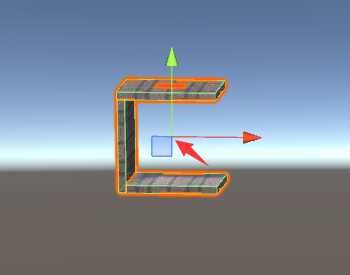多个物体的中心点：如果你选中了多个物体，那么这些物体整体的中心点在哪呢？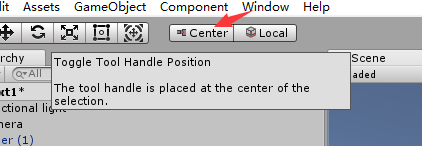Center：当选中多个模型时，模型组的中心点在所有物体中心点的最中间
Pivot：当选中多个模型时，模型组的中心点在你选中的最后一个物体身上
Center状态例子：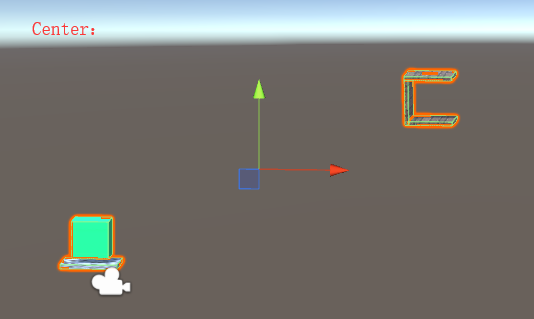如何改变模型中心点？创建一个空物体，创建父子关系，通过父物体来控制子物体即可间接改变物体中心点

例子如下：这个时候若要旋转右边的组合物体，就会以图中的中心点为轴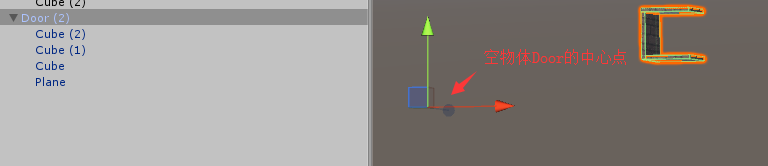（当然把中心点设在这里非常的不合理，所以在之后将其改成了组合物体的中心点）

二、按键控制物体旋转

Transform.Rotate(Vector3，float)：让物体沿Vector3方向旋转float度

using System.Collections;
using System.Collections.Generic;
using UnityEngine;
public class LRtoDoor : MonoBehaviour
{
private Transform myTransform;
private Rigidbody myRigidbody;
void Start()
{
myTransform = gameObject.GetComponent<Transform>();        //获取相应对象的引用
myRigidbody = gameObject.GetComponent<Rigidbody>();
}
void Update()
{
if (Input.GetKeyDown(KeyCode.Z))
myTransform.Rotate(Vector3.down, 90);           //按下Z键让物体沿世界坐标系前方旋转90°
if (Input.GetKeyDown(KeyCode.X))
myTransform.Rotate(Vector3.up, 90);
}
}


效果如下：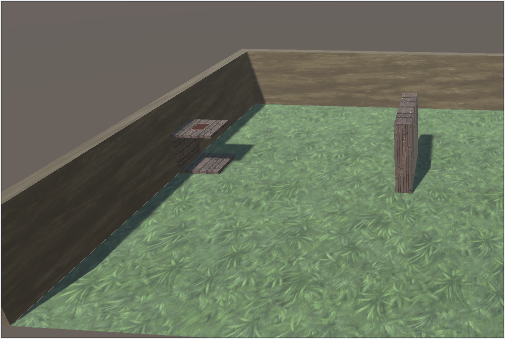展开全文Unity3D
• 当腕关节中心（关节4、5、6的旋转汇交的一点）的位置位于第一关节轴线的上方时，该位置称为机器人的顶部奇异。处于该位置时，首先第一关节的会有无穷多解；其次容易造成关节1和关节4瞬间旋转180°；最后还有更为...
• 小白欢迎评论，共同探讨，共同进步  此系列博文为程序小白记录笔记，以防... 按W使其为位移模式，然后按快捷键S（捕捉），鼠标放到上，相应轴线会变黄。  再移动轴线就可以了    使物体回归
• k-means算法有个很大的缺点，就是对孤立点敏感性太高，孤立点即是脱离群众的点，与众不同的点，即在显示中其他点不是抱在一团的点。 ...使用k-means算法运行，定义3个中心点： 可以看到...聚类
• // 先将旋转中心点平移到原点，同时也要平移待旋转点 X -= center.X; Y -= center.Y; // 设置当前点坐标为旋转后的点 set(X*cs - Y*sn, X*sn + Y*cs); // 将旋转后的点平移回去 X += center.X; Y += center....
• 当我们将模型导入到Unity3D中时，经常会遇到模型的中心点与物体中心点相差甚远的情况 这种情况通常会对我们的物体位置控制产生一些不利影响（当然这种设置也有其存在的好处……） 要解决类似问题，我们通常可以在...unity3d 模型
• 6串联关节机器人有三种奇点：腕部奇点，肩部奇点，肘部奇点。  1、腕部奇点发生在4和6重合(平行)时...1. 4和6产生奇异(wrist singularity) 下图中的六机器人，四和六相交（大部分机器人四...
• ## 西储大学轴承数据中心网站数据

万次阅读 多人点赞 2016-05-04 15:04:38
本文引自西储大学轴承数据中心网站\hspace{1cm}This website provides access to ball bearing test data for normal and faulty bearings. Experiments were conducted using a 2 hp Reliance Electric motor, and ...
• 图形化广泛应用于各种系统中，包括ESB的适配，业务流程的建模，工作流程的定制，数据交换系统的配置等等，这其中不可避免的需要用到各种图形符号来直观、清晰的表达抽象的内容。...　本文实现了一个获取矩形中心点
• 在我们使用OpenGL画的时候，默认的中心是在屏幕的中心，左右，上下，前后都是-1到1的区间，如果我们需要绘制大于这个区间的怎么办呢？ 方法一： 我们可以将所有的需要绘制的转化到-1到1的区间。 方法二： ...
• img = cv2.imread(pic_path) h, w = img.shape w表示横方向的长度，h表示纵方向的长度，一般我们表示坐标位置都是横轴为x，纵轴为y，所以中心点坐标应该表示为 (w/2, h/2) ...cv2
• 用一句话概括注册点和中心点的作用，那就是：注册点用来定位，中心点用来变形 当然，这句话不是非常准确，只是暂时先这么理解，下面会详细讲解。 认识注册点 每个元件都有一个注册点。在元件编辑窗口、或在...flash
• 1 对捕捉按钮右键，在捕捉设置里设置勾选启用约束，这样，移动时点被强制在某个轴向移动。 2 设置捕捉到顶点或端点或其他元素。 3 按F5，使在移动时强制在X轴向移动， 按F6，使在移动时强制在Y轴向移动， 按...
• 当我们对一个View设置缩放动画时，缩放点默认是该View的中心点。如果我们想改变缩放点位置，可以通过setPivotX(float pivotX)设置缩放点X的坐标，通过setPivotY(float pivotY)设置缩放点Y的坐标。 下面...

千次阅读 2020-07-16 14:58:11
• matplotlib 可视化 —— 移动坐标中心位置）
• 旋转方式有中心旋转、旋转。1. 罗德里格旋转公式 在三维旋转理论体系中，罗德里格旋转公式（根据欧林·罗德里格命名）是在给定转轴和旋转角度后，旋转一个向量的有效算法。如果v是在中的向量，k是转轴的单位向量...
• Point Util::Rotate(Point p, double angle, double x, double y, double z) //注意这里的（x,y,z）是单位化的旋转向量 {  double m;  //D3DXVec3Normalize();  //angle = 1.57;  double u = x;  ...
• 已知旋转后的坐标系xx'夹角a，yy'夹角b，zz'夹角c，x'y'z'为自然坐标系的，xyz为旋转后的坐标系，怎么求旋转角旋转？用一立方体表示，因为中心点在两斜对角上，是否可以用斜对角的变化来表示？
• 上面是一张比较复杂的图形，我们希望通过Halcon一系列的算子进行处理，查找到星点交叉处的中心点。mean_image (Image, ImageMean, 41, 41)将图片进行均值处理，让分离的星点模糊化，从而达到融合成一个区域的目的。 ...图片
• 1.描述 　上一篇提到了物体的坐标朝向，这一篇来说说物体的坐标中心点。单个物体 ，或者包含很多物体的父物体，我们希望 ...1.底端中心点 2 中间中心点 3 顶端中心点 2.代码如下 using System.C...unity...